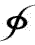a . . . Paradigm Shift !Understanding the Singularity of Reality, as described by mathematics and physics, begins with Infinity and seminal motion; and thus is found, the Conceptual Unit, CU, One, as manifested by said motion, that is heuristically described by the: Brunardot Theorem: c2 = 2v2 - s2. The Brunardot Theorem describes every ellipse; thus: the Brunardot Theorem is to an ellipse as Pythagoras' Theorem is to a right angle. And, when d - 2s = CU = 1, then, v = p2. The significance of the vector equaling the perigee squared is that the Conceptual Unit, One, is directly related to squares of dimensions and the ellipse, both of which are salient qualities of seminal motion; and, both qualities are omnipresent throughout Reality.